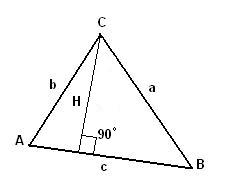# Altitude of a triangle

This online calculator computes the altitude length of a triangle, given the lengths of sides of a triangle.As usual, triangle sides are named a (side BC), b (side AC) and c (side AB).
The altitude of a triangle to side c can be found as:

where S - an area of a triangle, which can be found from three known sides using, for example, Hero's formula, see Heron's formula calculator#### Altitude of a triangle

Digits after the decimal point: 2
Altitude to edge c

URL copiado para a área de transferência